Courses

# Solved Examples - Solutions (Part - 2) Notes | EduRev

## JEE : Solved Examples - Solutions (Part - 2) Notes | EduRev

The document Solved Examples - Solutions (Part - 2) Notes | EduRev is a part of the JEE Course Chemistry for JEE.
All you need of JEE at this link: JEE

Example 50. Calculate normality of the mixture obtained by mixing 100ml of 0.1N HCl and 50ml of 0.25N NaOH solution.
(a) 0.0467 N
(b) 0.0367 N
(c) 0.0267 N
(d) 0.0167 N
Ans. (d)
Solution.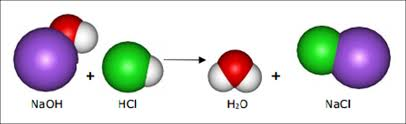Molal eq. of HCl = 100 × 0.1 = 10
Molal eq. of NaOH = 50 × 0.25 = 12.5
HCl and NaOH neutralize each other with equal eq.
Eq. of NaOH left = 12.5 - 10 = 2.5
Volume of new solution = 100 + 50 = 150 ml.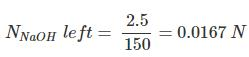Hence normality of the mixture obtained is 0.0167 N

Example 51. 300 ml 0.1 M HCl and 200 ml of 0.03M H2SO4 are mixed. Calculate the normality of the resulting mixture-
(a) 0.084 N
(b) 0.84 N
(c) 2.04 N
(d) 2.84 N
Ans. (a)
Solution.
For HCl For H2SO4
V1 = 300 ml V2 = 200 ml
N1 = M × Basicity N2 = M × Basicity
= 0.1 × 1 = 0.1 = 0.03 × 2 = 0.06
Normality of the mixture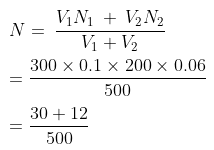= 0.084 N

Example 52. In what ratio should a 6.5 N HNO3 be diluted with water to get 3.5 N HNO3?
(a) 6 : 7
(b) 7 : 6
(c) 5 : 6
(d) 6 : 5
Ans. (b)
Solution.
N1V1 = N2V2
6.5 V1 = 3.5 (V1 x)
6.5 V1 = 3.5 V1 3.5 x
3 V1 = 3.5 x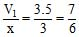Example 53. Calculate the amount of each in the following solutions -
(i) 150 ml of N/7 H2SO4
(ii) 250 ml of 0.2M NaHCO3
(iii) 400 ml of N/10 Na2CO3
(iv) 1052 g of 1 m KOH.
(a) 52g, 2.12g, 4.2g, 1.05g
(b) 1.05g, 4.2g, 2.12g, 52g
(c) 1.05g, 2.12g, 52g, 4.2g
(d) 4.2g, 2.12g, 1.05g, 52g
Ans. (b)
Solution.
(i) Eq. wt. of H2SO4Amount of H2SO4 per litre (strength) = Normality × Eq. wt. = × 49 = 7 g/litre
Amount in 150 ml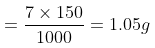(ii) Molecular wt. of
NaHCO3 = 23 1 12 48 = 84
Amount of NaHCO3 required to produce 1000 c.c. of one molar solution = 84 g
Amount present per litre in 0.2 M solution = 84 × 0.2 = 16.8 g
Amount present in 250 c.c.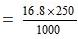= 4.2 g
(iii) Equivalent weight of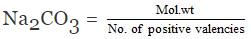= 53
Amount of Na2CO3 = Normality × Eq. wt. = × 53 = 5.3 g/litre
Amount present in 400 c.c.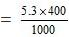= 2.12 g
(iv) We know that 1 molal solution of a substance contains 1000 g of solvent.
Wt. of KOH in 1052 g of 1 m KOH solution = 1052 - 1000 = 52 g

Example 54. How many kilograms of wet NaOH containing 12% water are required to prepare 60 litres of 0.50 N solution ?
(a) 1.36 kg
(b) 1.50 kg
(c) 2.40 gm
(d) 3.16 kg
Ans. (a)
Solution.
One litre of 0.50 N NaOH contains = 0.50 × 40g = 20 g = 0.020 kg
60 litres of 0.50 N NaOH contain
= 0.020 × 60 kg = 1.20 kg NaOH
Since the given NaOH contains 12% water, the amount of pure NaOH in 100 kg of the given NaOH = 100 - 12 = 88 kg
Thus 88 kg of pure NaOH is present in 100 kg wet NaOH
1.20 kg of pure NaOH is present in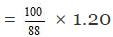= 1.36 kg wet NaOH

Example 55. Calculate the vapour pressure of a solution at 1000C containing 3g of cane sugar in 33g of water. (At wt. C = 12 , H = 1 , O = 16)
(a) 760 mm
(b) 756.90 mm
(c) 758.30 mm
(d) None
Ans. (b)
Solution.
Vapour pressure of pure water (solvent) at 1000C, p° = 760 mm.
Vapour pressure of solution, p = ?
Wt. of solvent, W = 33g
Wt. of solute, w = 3g
Mol. wt. of water (H2O), M = 18
Mol. wt. of sugar (C12H22O11),
m = (12 × 12) + (22 × 1) + (11 × 16) = 342
According to Raoult's law,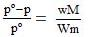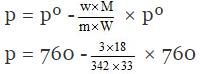(pº for H2O = 760 mm)
= 760 - 3.19 = 756.90 mm

Example 56Osmotic pressure of a sugar solution at 24°C is 2.5 atmospheres. Determine the concentration of the solution in gm mole per litre.
(a) 0.0821 moles/litre
(b) 1.082 moles/litre
(c) 0.1025 moles/litre
(d) 0.0827 moles/litre
Ans. (c)
Solution.
Here it is given that
p = 2.5 atm, T = 24 273 = 297K, S = 0.0821 lit. atm. deg-1 mol-1, C = ?
We know that p = CST
or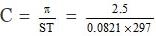= 0.1025 moles/litre

Example 57. Twenty grams of a substance were dissolved in 500 ml. of water and the osmotic pressure of the solution was found to be 600 mm of mercury at 15ºC. Determine the molecular weight of the substance-
(a) 1120
(b) 1198
(c) 1200
(d) None of these
Ans. (c)
Solution.
Here it is given that
w = 20 gm ; V = 500 ml.
= 500/1000 = 0.5 litre
p = 600 mm = 600/760  atm;
T = 15 273 = 2880A
m = ?
According to Van't Hoff equation,
pV = nST pV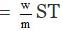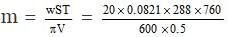= 1198

Example 58. Blood plasma has the following composition (milli-equivalents per litre). Calculate its osmotic pressure at 370C.
Na  = 138 , Ca = 5.2, K  = 4.5, Mg = 2.0 , Cl¯ = 105, HCO3¯ = 25, PO43-= 2.2 , SO42-= 0.5,
Proteins = 16, Others = 1.0
(a) 7.47 atm
(b) 7.30 atm
(c) 7.29 atm
(d) 7.40 atm
Ans. (a)
Solution.
Since for calculating osmotic pressure we require millimoles/litre therefore
Na+= 138 Ca2+ = 5.2/2 = 2.6, K = 4.5,
Mg2+ = 2.0/2 = 1.0 , Cl¯ = 105,
HCO3¯ = 24,PO43-=  2.2/3 = 0.73,
SO42-= 0.5/2 = 0.25 , Proteins = 16,
others = 1.0
Total = 294.18 millimoles/litre = 294.18/1000
= 0.294 moles/litre
Now since p = CST
= 0.294 × 0.0821 × .310 = 7.47 atm

Example 59. 0.15g of a substance dissolved in 15g of solvent boiled at a temperature higher by 0.2160C than that of the pure solvent. Calculate the molecular weight of the substance. Molal elevation constant for the solvent is 2.160C.
(a) 216
(b) 100
(c) 178
(d) None of these
Ans. (b)
Solution.
Here it is given that
w = 0.15 g, DTb = 0.2160C
W = 15g Kb = 2.160C
m = ?
Substituting values in the expression,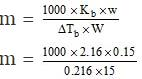= 100

Example 60. A solution of 0.450 gm of urea (mol. wt 60) in 22.5 g of water showed 0.1700C of elevation in boiling point. Calculate the molal elevation constant of water-
(a) 0.17ºC
(b) 0.45ºC
(c) 0.51ºC
(d) 0.30ºC
Ans. (c)
Solution.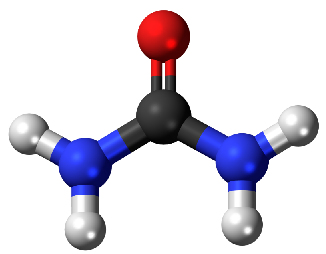Fig: Structure of urea

Wt. of solute, w = 0.450 g
Wt. of solvent, W = 22.5 g
Mol. wt of solute, m = 60
Molal elevation constant Kb = ?
Boiling point elevation, DTb = 0.1700C
Substituting these values in the equation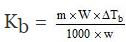=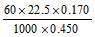= 0.510C

Example 61. Calculate the boiling point of a solution containing 0.45g of camphor (mol. wt. 152) dissolved in 35.4g of acetone (b.p. 56.30C); Kb per 100 gm of acetone is 17.20C.
(a) 56.446°C
(b) 52.401°C
(c) 56.146°C
(d) 50.464°C
Ans. (a)
Solution.
Here it is given that
w = 0.45 g, W = 35.4, m = 152,
Kb = 17.2 per 100gm
Now we know that ΔTb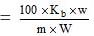(Note that this is expression when Kb is given per 100g of the solvent)
Substituting the values in the above expression.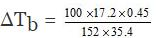= 0.1460C
Now we know that
B.P. of solution (T) - B.P. of solvent (T0) = DT
B.P. of solution (T) = B.P. of solvent(T0) DT
Hence B.P. of solution = 56.3 0.146
= 56.4460C

Example 62. The freezing point of 0.2 molal K2SO4 is _1.1ºC. Calculate Van't Haff factor and percentage degree of dissociation of K2SO4. Kf for water is 1.86º
(a) 97.5
(b) 90.75
(c) 105.5
(d) 85.75
Ans. (a)
Solution.
ΔTf = freezing point of water -freezing point of solution = 0º C - (1.1º C) = 1.1º
We know that,
ΔTf = i × Kf × m
1.1 = i × 1.86 × 0.2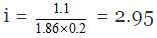But we know
i = 1 (n - 1) a
2.95 = 1 (3 - 1) a = 1 2a
a = 0.975
Van't Haff factor (i) = 2.95
Degree of dissociation = 0.975
Percentage degree of dissociation = 97.5

Offer running on EduRev: Apply code STAYHOME200 to get INR 200 off on our premium plan EduRev Infinity!

## Chemistry for JEE

223 videos|446 docs|334 tests

,

,

,

,

,

,

,

,

,

,

,

,

,

,

,

,

,

,

,

,

,

;